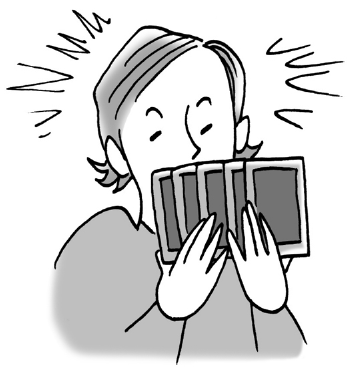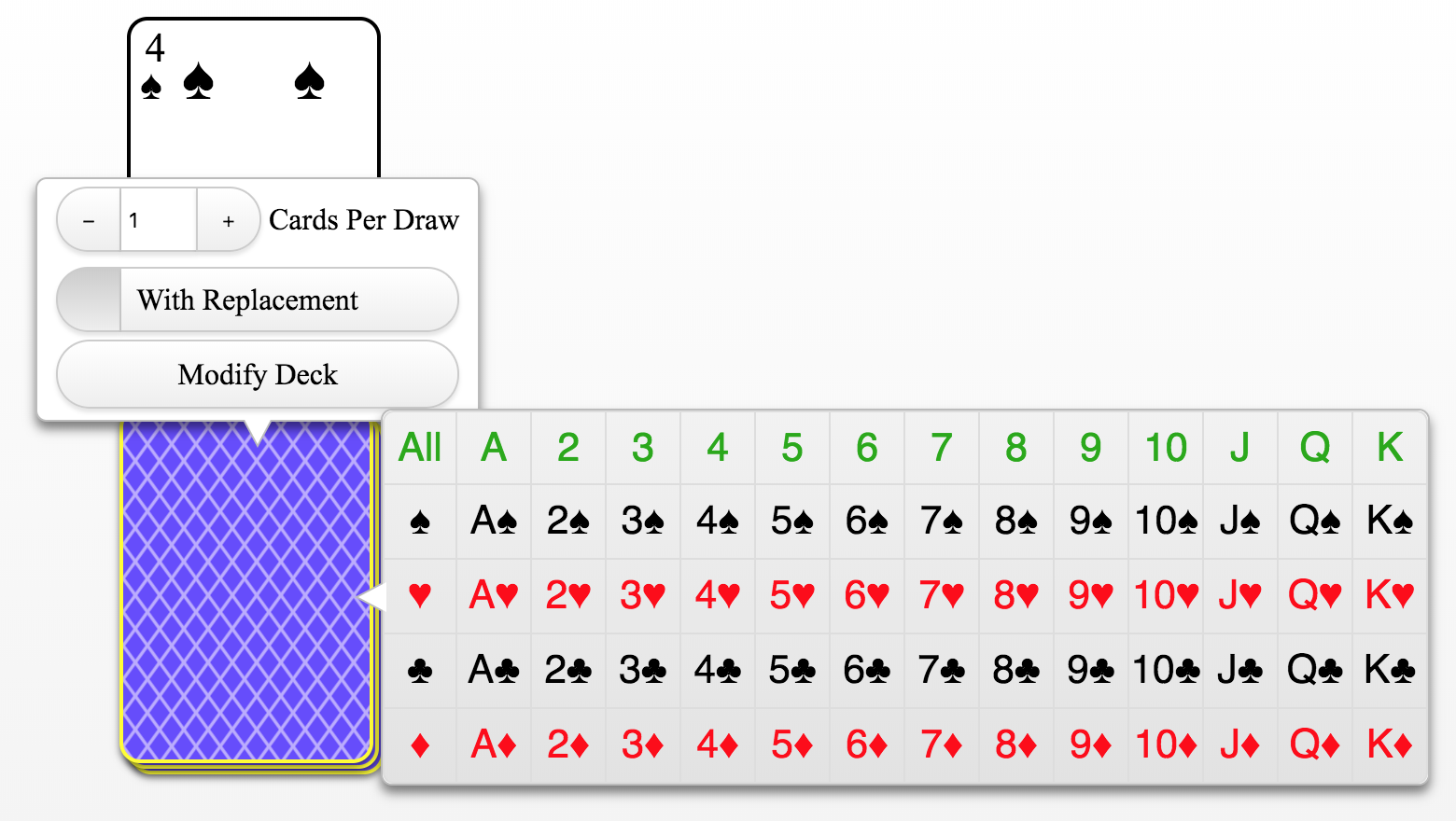### Home > CCG > Chapter 4 > Lesson 4.2.5 > Problem4-116

4-116.Avery has been learning to play some new card games and is curious about the probabilities of being dealt different cards from a standard $52$-card deck. Help him figure out the probabilities listed below.

1. What are P(king), P(queen), and P(club)?

How many kings are in a deck of cards? Queens? Clubs?2. What is $P(\text{king or club})$? How does your answer relate to the probabilities you calculated in part (a)?

Use the Addition Rule to eliminate P(king and club).

$P(\text{king or club) }= \frac{16}{52}$

3. What is P(king or queen)? Again, how does your answer relate to the probabilities you calculated in part (a)?